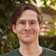# Leetcode - Episode 6 - Progressively Harder (3x E)

January 06, 2019

I’ve been going through the problems roughly sorted by their acceptance rate: higher `->` lower. Today, the difficulty is slowly creeping up. I’m looking forward to tackling some Mediums soon.

### 500. Keyboard Row

Problem: Given a list of `words`, return only those that can be typed using one keyboard row

This was a neat problem to solve. At one point, I was `lower()`ing the words to account for capitalization but simply adding capital letters to the Sets decreased the runtime by ~13%.

``````class Solution(object):
def findWords(self, words):
"""
:type words: List[str]
:rtype: List[str]
"""
rows = [set('qwertyuiopQWERTYUIOP'),
set('asdfghjklASDFGHJKL'),
set('zxcvbnmZXCVBNM')]
one_row_words = []

for word in words:
for row in rows:
if word in row:
if all(char in row for char in word[1:]):
one_row_words.append(word)
break
return one_row_words``````

I made sure to break once the word’s row had been found to save on loop cycles over ‘dead’ words.

Runtime complexity: `O(n)`.

Spacetime complexity: `O(1)`.

### 937. Reorder Log Files

Problem: Given an array of `logs` with alphanumeric identifiers at the start, sort them so that letter-only logs come before digit-only logs.

I’ve added in some comments where there are specific rules but I recommend checking their description as this one’s problem statement is harder to understand than any solution.

This was a fun one to solve.

``````class Solution(object):
def reorderLogFiles(self, logs):
"""
:type logs: List[str]
:rtype: List[str]
"""
letter_logs = []
digit_logs = []
log = log.split()
# check ascii value to determine digit or letter log
if ord(log) < 66:
# the digit-logs should be put in their original order
digit_logs.append(log)
else:
letter_logs.append(log)

# the letter-logs are ordered lexicographically ignoring identifier,
# with the identifier used in case of ties
letter_logs.sort(key=lambda x: x[1:] + x[0:1])

I’m getting a little better with Python generators. I really like how they feel to use, and how they affect the structure of these programs.

Runtime complexity: Separate logs `O(n)`, sort letter logs `O(n log n)`, join logs `O(n)`. Ergo: `O(n)`.

Spacetime complexity: `O(n)`.

### 136. Single Number

Problem: Given an array `N` of integers, numbers all appear twice except for one. Return that integer.

I knew this could be XORed but I couldn’t remember so implemented it with a Set and made a note to check after.

``````class Solution(object):
def singleNumber(self, nums):
"""
:type nums: List[int]
:rtype: int
"""
nums_set = set()
for i in nums:
if i in nums_set:
nums_set.remove(i)
else:
for i in nums_set:
return i``````

Runtime complexity: `O(n)`.

Space complexity: `O(n)`.

And now without that extra space, using XOR.

``````class Solution(object):
def singleNumber(self, nums):
"""
:type nums: List[int]
:rtype: int
"""
result = 0
for i in nums:
result ^= i
return result``````

Runtime complexity: `O(n)`.

Space complexity: `-`.

See you next time.